IMO Shortlist 2014 problem A2

Kvaliteta:
Avg: 5,0
Težina:
Avg: 6,0

Define the function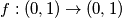$f : (0, 1) \to (0, 1)$ by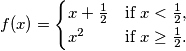Let$a$ and$b$ be two real numbers such that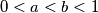$0 < a < b < 1$. We define the sequences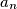$a_n$ and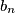$b_n$ by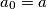$a_0 = a$,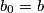$b_0 = b$, and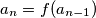$a_n = f(a_{n-1})$,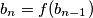$b_n = f(b_{n-1})$ for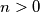$n > 0$. Show that there exists a positive integer$n$ such that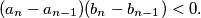(Denmark)

Izvor: https://www.imo-official.org/problems/IMO2014SL.pdf